# Cumulative Default Probability

## Definition

The term Cumulative Default Probability is used in the context of multi-period credit risk analysis to denote the likelihood that a legal entity is observed to have experienced a Credit Event up to a particular timepoint.

The cumulative default probability can be considered as the primary representation of the Credit Curve as a set of non-decreasing probabilities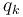$q_k$

## Relationships with related measures

• In terms of the Incremental Default Probability we have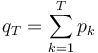$q_T = \sum_{k=1}^{T}{p_k}$ where we denote with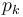$p_k$ the incremental default probability during time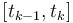$[t_{k-1}, t_k]$
• In terms of the Marginal Default Probability we have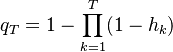$q_T = 1 - \prod_{k=1}^{T} (1 - h_k)$ where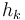$h_k$ is the marginal default probability during period$[t_{k-1}, t_k]$. The marginal default probability is also denoted the Hazard Rate
• In terms of the Survival Probability we have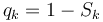$q_k = 1 - S_k$ where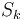$S_k$ is the survival probability up to point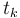$t_k$